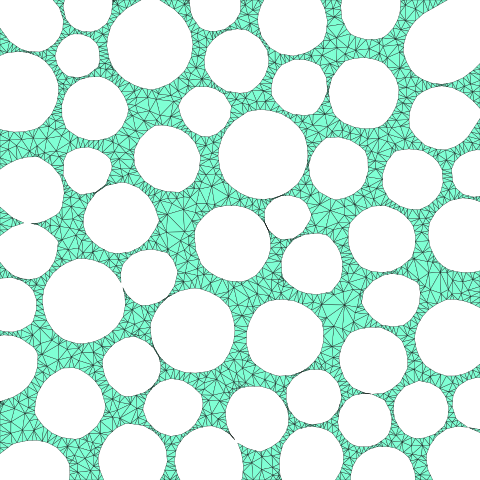# Foam¶

In this example, a foam microstructure is generated by first tesselating the voids, then adding foam material to the edges between the voids.

## Python Script¶

The basename for this file is `foam.py`. The file can be run using this command:

```microstructpy --demo=foam.py
```

The full text of the script is:

```import os

import numpy as np
import scipy.stats
from matplotlib import pyplot as plt

import microstructpy as msp

def main():
# Create Directory
dirname = os.path.join(os.path.dirname(__file__), 'foam')
if not os.path.exists(dirname):
os.makedirs(dirname)

# Define Domain
domain = msp.geometry.Square(side_length=8)

# Create Void Tessellation
void_mat = {'material_type': 'void',
'shape': 'circle',
'size': scipy.stats.lognorm(scale=1, s=0.2)
}

void_a = 0.7 * domain.area
void_seeds = msp.seeding.SeedList.from_info(void_mat, void_a)
void_seeds.position(domain, rtol=0.03, verbose=True)
void_tess = msp.meshing.PolyMesh.from_seeds(void_seeds, domain)

foam_mat = {'material_type': 'amorphous',
'shape': 'circle',
'size': scipy.stats.lognorm(scale=0.15, s=0.1)
}

foam_a = 0.15 * domain.area
foam_seeds = msp.seeding.SeedList.from_info(foam_mat, foam_a)
inds = np.flip(np.argsort([s.volume for s in foam_seeds]))
foam_seeds = foam_seeds[inds]

bkdwns = np.array([s.breakdown for s in foam_seeds])
np.random.seed(0)
for i, seed in enumerate(foam_seeds):
if i == 0:
trial_pt = trial_position(void_tess)
else:
r = seed.geometry.r
check_bkdwns = bkdwns[:i]
good_pt = False
while not good_pt:
trial_pt = trial_position(void_tess)
good_pt = check_pt(trial_pt, r, check_bkdwns)

seed.position = trial_pt
bkdwns[i] = seed.breakdown
seed.phase = 1

# Combine Results
materials = [void_mat, foam_mat]
seeds = void_seeds + foam_seeds
pmesh = msp.meshing.PolyMesh.from_seeds(seeds, domain)

# Triangular Mesh
tmesh = msp.meshing.TriMesh.from_polymesh(pmesh,
materials,
min_angle=20,
max_edge_length=0.1)

# Plot
tmesh.plot(facecolor='aquamarine',
edgecolor='k',
linewidth=0.2)

plt.gca().set_position([0, 0, 1, 1])
plt.axis('image')
plt.gca().set_axis_off()
plt.gca().get_xaxis().set_visible(False)
plt.gca().get_yaxis().set_visible(False)

xlim, ylim = domain.limits
plt.axis([xlim, xlim, ylim, ylim])

for ext in ['png', 'pdf']:
fname = os.path.join(dirname, 'trimesh.' + ext)

def pick_edge(void_tess):
f_neighs = void_tess.facet_neighbors
i = -1
neighs = [-1, -1]
while any([n < 0 for n in neighs]):
i = np.random.randint(len(f_neighs))
neighs = f_neighs[i]
facet = void_tess.facets[i]
j = np.random.randint(len(facet))
kp1 = facet[j]
kp2 = facet[j - 1]
return kp1, kp2

def trial_position(void_tess):
kp1, kp2 = pick_edge(void_tess)
pt1 = void_tess.points[kp1]
pt2 = void_tess.points[kp2]

f = np.random.rand()
return [f * x1 + (1 - f) * x2 for x1, x2 in zip(pt1, pt2)]

def check_pt(point, r, breakdowns):
pts = breakdowns[:, :-1]
rads = breakdowns[:, -1]

rel_pos = pts - point
dist = np.sqrt(np.sum(rel_pos * rel_pos, axis=1))
min_dist = rads + r - 0.3 * np.minimum(rads, r)
return np.all(dist > min_dist)

if __name__ == '__main__':
main()
```

## Domain¶

The domain of the microstructure is a `Square`. Without arguments, the square’s center is (0, 0) and side length is 15.

## Seeds¶

Initially, the seed list is entirely voids following a lognormal size distribution. These are then tessellated to determine the boundaries between the voids. These voids are generated to fill 70% of the domain and are positioned with a custom `rtol` value of 3%. This ensures that most of the voids do not connect with each other and that the foam seeds positioned along the edges do not become consumed by the void cells.

Next, foam seeds are added to the edges between voids. These seeds are given a size distribution, however there are no foam grains since the material is amorphous. Once the foam seeds are positioned in the domain, the lists of void and foam seeds are combined into a single seed list.

## Polygon Mesh¶

A polygon mesh is created from the list of seed points using the `from_seeds()` class method.

## Triangular Mesh¶

A triangular mesh is created from the polygonal mesh using the `from_polymesh()` class method. The optional `phases` parameter is used in this case since the mesh contains non-crystalline materials. Additionally, the minimum interior angle of the mesh elements is set to 20 to ensure good mesh quality and the maximum edge length is set to increase mesh resolution near the voids.

## Plotting¶

The triangular mesh in this example is plotted in aquamarine, one of several named colors in matplotlib. Next, the axes are turned off and the limits are set to equal the bounds of the domain. Finally, the triangular mesh is saved as a PNG and as a PDF, with the resulting plot shown in Fig. 22.Fig. 22 Foam example - triangular mesh.¶